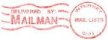McStas: Saxs_spheres

# The `Saxs_spheres` Component

Release: McXtrace 1.1 Sample for Small Angle X-ray Scattering - hard spheres in thin solution, mono disperse.

## Identification

• Site:
• Author: E. B. Knudsen, P. Willendrup, K. Lefmann, L. Arleth
• Origin: DTU Fysik
• Date: 28.10.2010

## Description

```Sample for use in a SAXS instrument, models hard, monodisperse spheres in thin solution.
The shape of the sample may be a filled box with dimensions
xwidth, yheight, zdepth, a cylinder with dimensions radius and yheight,
a filled sphere with radius R.

Example: Saxs_spheres(R = 20, Phi = 1e-3, Delta_rho = 0.6, sigma_abs = 50, xwidth=0.01, yheight=0.01, zdepth=0.005)
```

## Input parameters

Parameters in boldface are required; the others are optional.
 Name Unit Description Default sphere_mtrl Material datafile from which to find absorption. If none is given absorption is neglected. "" R Radius of scattering hard spheres (AA) 100 Phi Particle volume fraction (1) 1e-3 Delta_rho Excess scattering length density (fm/AA^3) 0.6 xwidth horiz. dimension of sample, as a width (m) 0 yheight vert . dimension of sample, as a height for cylinder/box (m) 0 zdepth depth of sample (m) 0 radius Outer radius of sample in (x,z) plane for cylinder/sphere [m] 0 target_x 0 target_y position of target to focus at [m] 0 target_z 6 target_index Relative index of component to focus at, e.g. next is +1  0 focus_xw horiz. dimension of a rectangular area [m] 0 focus_yh vert. dimension of a rectangular area [m] 0 focus_aw horiz. angular dimension of a rectangular area [deg] 0 focus_ah vert. angular dimension of a rectangular area [deg] 0 focus_r Detector (disk-shaped) radius (m) 0 mu_c 5 Column of the datafiel which contains absorption coefficients. 0

## Output parameters

Parameters in boldface are required; the others are optional.
 Name Unit Description Default